## GATE 1993

 Question 1

The eigen vector(s) of the matrixis (are)

 A (0,0,α ) B (α,0,0) C (0,0,1) D (0,α,0) E Both B and D
Engineering-Mathematics       Linear-Algebra
Question 1 Explanation:
Since, the given matrix is an upper triangular one, all eigen values are A. And hence A - λI = A.
So the question as has
(A - λI)X = 0
AX = 0What x1, x2, x3 are suitable?
Which means:
x1 times column 1 + x2 times column 2 + x3 times column 3 = zero vector
Since α is not equal to zero, so x3 must be necessarily zero to get zero vector.
Hence, only (B) and (D) satisfies.
 Question 2

The differential equation
d2y/dx2 + dy/dx + siny = 0 is:

 A linear B non-linear C homogeneous D of degree two
Engineering-Mathematics       Calculus
Question 2 Explanation:
Note: Out of syllabus.
d2y/dx2 + dy/dx + siny = 0
In this DE, degree is 1 then this represent linear equation.
 Question 3

Simpson’s rule for integration gives exact result when f(x) is a polynomial of degree

 A 1 B 2 C 3 D 4
Engineering-Mathematics       Simphson\'s-Rule
Question 3 Explanation:
Note: Out of syllabus.
 Question 4

Which of the following is (are) valid FORTRAN 77 statement(s)?

 A DO 13 I = 1 B A = DIM ***7 C READ = 15.0 D GO TO 3 = 10
Computer-Organization       FORTRAN
Question 4 Explanation:
Note: Out of syllabus.
 Question 5

Fourier series of the periodic function (period 2π) defined by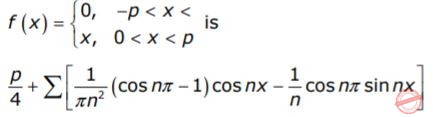But putting x = π, we get the sum of the series.A π2/4 B π2/6 C π2/8 D π2/12
Engineering-Mathematics       Calculus
Question 5 Explanation:
Note: Out of syllabus.
 Question 6

Which of the following improper integrals is (are) convergent?

 AB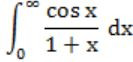CD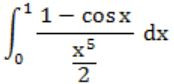Engineering-Mathematics       Calculus
 Question 7

The function f(x,y) = x2y - 3xy + 2y + x has

 A no local extremum B one local minimum but no local maximum C one local maximum but no local minimum D one local minimum and one local maximum
Engineering-Mathematics       Functions
Question 7 Explanation:
Note: Out of syllabus.
 Question 8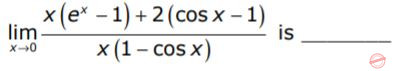A 1
Engineering-Mathematics       Calculus
Question 8 Explanation:
Since the given expression is in 0/0 form, so we can apply L-Hospital rule.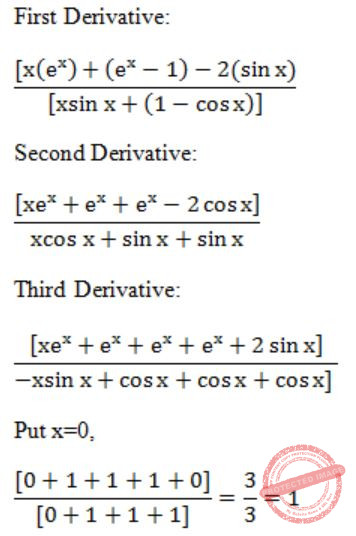Question 9

The radius of convergence of the power seriesA Out of syllabus.
Engineering-Mathematics       Calculus
 Question 10

If the linear velocityis given byThe angular velocityat the point (1, 1, -1) is ________

 A Out of syllabus.
Engineering-Mathematics       Vectors
 Question 11

Given the differential equation, y′ = x − y with the initial condition y(0) = 0. The value of y(0.1) calculated numerically upto the third place of decimal by the second order Runga-Kutta method with step size h = 0.1 is ________

 A Out of syllabus.
Engineering-Mathematics       R-K-Method
 Question 12

For X = 4.0, the value of I in the FORTRAN 77 statement
1 = -2**2 + 5.0*X/X*3 + 3/4 is _______

 A Out of syllabus.
Engineering-Mathematics       FORTRAN
 Question 13

The value of the double integral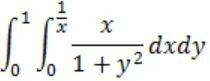is

 A 1/3
Engineering-Mathematics       Calculus
Question 13 Explanation:Question 14

If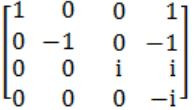the matrix A4, calculated by the use of Cayley-Hamilton theorem or otherwise, is _________

 A A4 = I
Engineering-Mathematics       Linear-Algebra
Question 14 Explanation:
Let λ be eigen value, then characteristic equation will be
(1-λ) (-1-λ) (i-λ) (-i-λ)
= (λ2-1) (λ2+1)
= λ4-1
Characteristic equation is λ4-1 = 0.
According to Cayley-Hamilton theorem, every matrix satisfies its own characteristic equation, so
A4 = I
 Question 15

Givenand S the surface of a unit cube with one corner at the origin and edges parallel to the coordinate axes, the value of integralA Out of syllabus.
Engineering-Mathematics       Vector Algebra
There are 15 questions to complete.

Register Now